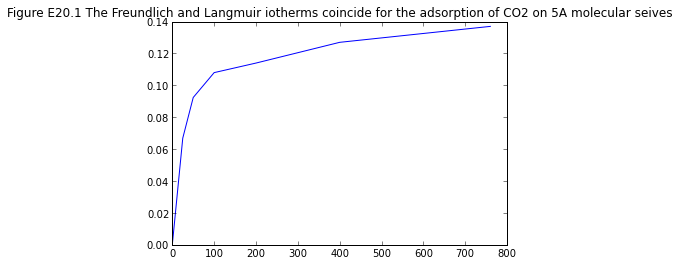# Chapter 20 : Liquid and Gases in Equilibrium with Solids¶

### Example 20.1 Page no. 594¶

In :
%matplotlib inline

from matplotlib import pyplot as plt

# Variables
p_CO2 = [0,25,50,100,200,400,760] ;			# Values of partial pressure of CO2 - [mm Hg]
y = [0,6.69*10**-2,9.24*10**-2,0.108,0.114,0.127,0.137] ;			# adsorption of CO2 -[g adorbed / g seives]

# Results
plt.plot(p_CO2,y);
plt.title('Figure E20.1 The Freundlich and Langmuir iotherms coincide for the adsorption of CO2 on 5A molecular seives');
plt.show()

Populating the interactive namespace from numpy and matplotlib### Example 20.2 page no. 596¶

In :
# Variables
G = 1000.0 ;			# Volume of solution - [L]
S_ad = 1.56 ;			# amount of Steptomycin adsorbed per gram resin-[g strep./g resin]
cn_S = 6. ;			# Concentration of streptomycin solution-[g/L]

# Calculations
# Assume equilibrium occurs so that total(max) amount of streptomycin is adsorbed
max_S = cn_S*G ;			# Maximum streptomycin adsorbed-[g]
#Use streptomycin balance to get amount of resin required
R = max_S/S_ad ;			#Amount of resin required to adsorb required amount of streptomycin

# Results
print 'Amount of resin required to adsorb required amount of streptomycin is %.0f g . '%R

Amount of resin required to adsorb required amount of streptomycin is 3846 g .


### Example 20.3 page no. 596¶

In :
# Variables
G = 1000. ;			# Volume of solution - [L]
x = [19.2,17.2,12.6,8.6,3.4,1.4]  ;			# concentration of solute- [g/L]
ac = [0,0.01,0.04,0.08,0.20,0.40]  ;			# Activated charcoal added-[g/1000g sol]
# Assume all concentration can be treated as g solute/1000 g sol.

# Calculations
y2 = (x-x)/ac  ;			# -[ g solute/g carbon]
y3 = (x-x)/ac  ;			# -[ g solute/g carbon]
y4 = (x-x)/ac  ;			# -[ g solute/g carbon]
y5 = (x-x)/ac  ;			# -[ g solute/g carbon]
y6 = (x-x)/ac  ;			# -[ g solute/g carbon]

# Use polymath to get Freundlich isotherm to bo y= 37.919*x**(0.583)
y = 37.919*x**(0.583) ;			#From Freundlich isotherm
A_by_G = (x-x)/y ;			#Minimum mss of activated carbon required- [g carbon/1000 g sol.]

# Results
print 'Minimum mass of activated carbon required is %.2f g carbon/1000 g sol.  '%A_by_G

Minimum mass of activated carbon required is 0.39 g carbon/1000 g sol.

In [ ]: